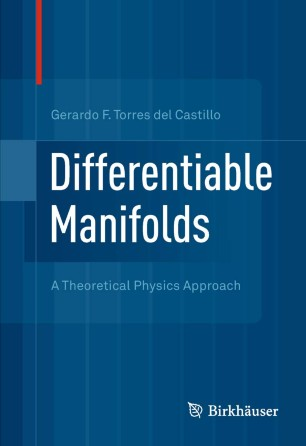# Differentiable Manifolds

## A Theoretical Physics Approach

• Gerardo F. Torres del CastilloTextbook

1. Front Matter
Pages I-VIII
2. Gerardo F. Torres del Castillo
Pages 1-28
3. Gerardo F. Torres del Castillo
Pages 29-48
4. Gerardo F. Torres del Castillo
Pages 49-65
5. Gerardo F. Torres del Castillo
Pages 67-91
6. Gerardo F. Torres del Castillo
Pages 93-114
7. Gerardo F. Torres del Castillo
Pages 115-160
8. Gerardo F. Torres del Castillo
Pages 161-200
9. Gerardo F. Torres del Castillo
Pages 201-253
10. Back Matter
Pages 255-275

### Introduction

This textbook gives a concise introduction to the theory of differentiable manifolds, focusing on their applications to differential equations, differential geometry, and Hamiltonian mechanics.

The work’s first three chapters introduce the basic concepts of the theory, such as differentiable maps, tangent vectors, vector and tensor fields, differential forms, local one-parameter groups of diffeomorphisms, and Lie derivatives. These tools are subsequently employed in the study of differential equations (Chapter 4), connections (Chapter 5), Riemannian manifolds (Chapter 6), Lie groups (Chapter 7), and Hamiltonian mechanics (Chapter 8). Throughout, the book contains examples, worked out in detail, as well as exercises intended to show how the formalism is applied to actual computations and to emphasize the connections among various areas of mathematics.

Differentiable Manifolds is addressed to advanced undergraduate or beginning graduate students in mathematics or physics. Prerequisites include multivariable calculus, linear algebra, differential equations, and (for the last chapter) a basic knowledge of analytical mechanics.

### Keywords

Euler equations Hamiltonian mechanics Lie derivatives Riemannian manifolds differentiable manifolds differential forms time-dependent formalism

#### Authors and affiliations

• Gerardo F. Torres del Castillo
• 1